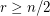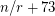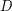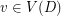# Caccetta-Häggkvist Conjecture

 Importance: Outstanding ✭✭✭✭
 Author(s): Caccetta, L. Häggkvist, Roland
 Subject: Graph Theory » Directed Graphs
 Keywords:
 Posted by: fhavet on: February 28th, 2013
Conjecture   Every simple digraph of orderwith minimum outdegree at leasthas a cycle with length at mostIt is one of the most famous conjectures in graph theory. It has many alternative formulations and lots of work have been done around it. Many interesting conjectures are related to it. See [Sul]. It is in particular implied by a conjecture of Thomassé and Hoàng-Reed Conjecture.

The Caccetta-Häggkvist Conjecture is a generalization of an earlier conjecture of Behzad, Chartrand, and Wall, who conjectured it only for diregular digraphs. Caccetta-H äggkvist Conjecture has been proved forby Shen [She1]. Forit is trivial. But already for, it is still open as well as Behzad-Chartrand-Wall Conjecture

Conjecture   Every simple-vertex digraph with minimum outdegree at leastand minimum indegree at leasthas a cycle with length at most.

This conjecture would be implied by Seymour's Second Neighbourhood Conjecure.

Shen [She2] also proved the following approximate version.

Theorem   Every simple digraph of orderwith minimum outdegree at leasthas a cycle with length at most.

Bollobás and Scott [BS] proposed a weighted version of the Caccetta-Häggkvist Conjecture.

Conjecture   Letbe a weight function on the arcs of a digraph. Ifandfor all, then there is a directed cycle inof total weight at least 1.

They gave a nice proof that there is a directed path of total weight at least 1.

## Bibliography

[BCW] M. Behzad, G. Chartrand, and C. Wall. On minimal regular digraphs with given girth. Fundamenta Mathematicae, 69:227–231, 1970.

[BS] B. Bollobás and A. D. Scott, A proof of a conjecture of {B}ondy concerning paths in weighted digraphs. J. Combin. Theory Ser. B, 66:283-292, 1996.

*[CH] L. Caccetta and R. Häggkvist. On minimal digraphs with given girth. Congressus Numerantium, XXI, 1978

[She1J. Shen. On the girth of digraphs. Discrete Math, 211(1-3):167–181, 2000.

[She2] J. Shen. On the Caccetta-Häggkvist conjecture. Graphs and Combinatorics, 18(3):645–654, 2002.

[Sul] Blair D. Sullivan: A Summary of Problems and Results related to the Caccetta-Häggkvist Conjecture

* indicates original appearance(s) of problem.